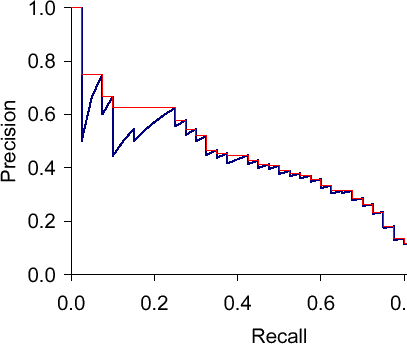# Precision, Recall and F-measure

In the last post we discussed accuracy, a straightforward method of calculating the performance of a classification system. Using accuracy is fine when the classes are of equal size, but this is often not the case in real world tasks. In such cases the very large number of true negatives outweighs the number of true positives in the evaluation so that accuracy will always be artificially high.

Luckily there are performance measures that ignore the number of true negatives. Two frequently used measures are precision and recall. Precision P indicates how many of the items that we have identified as positives are really positives. In other words, how precise have we been in our identification. How many of those that we think are X, really are X. Formally, this means that we divide the number of true positives by the number of all identified positives (true and false):$P = TP/(TP+FP)$

Recall R indicates how many of the real positives we have found. So from all of the positive items that are there, how many did we manage to identify. In other words, how exhaustive we were. Formally, this means that we divide the number of true positives by the number of all existing positives (true positives and false negatives):$R = TP/(TP+FN)$

For our example from the last post, precision and recall are as follows:$P = 1/(1+3) = 1/4 = 0.25$$R = 1/(1+2) = 1/3 = 0.33$

It is easy to get a recall of 100%. We just say for everything that it is a positive. But as this will probably not the case (or else we have a really easy dataset to classify!), this approach will give us a really low precision. On the other hand, we can usually get a high precision if we only classify as positive one single item that we are really, really sure about. But if we do that, recall will be low, as there will be more than one item in the dataset to be classified (or else it is not a very meaningful set).

So recall and precision are in a sort of balance. The F1 score or F1 measure is a way of putting the two of them together to produce one single number. Formally it calculates the harmonic mean of the two numbers and weights the two of them with the same importance (there are other variants that put more importance on one of them):$F_1 = (2 \cdot P \cdot R)/(P + R)$

Using the values for precision and recall for our example, F1 is:$F_1 = (2 \cdot 0.25 \cdot 0.33)/(0.25 + 0.33) = 0.165 / 0.58 = 0.28$

Intuitively, F1 is between the two values of precision and recall, but closer to the lower of the two. In other words, it penalizes if we concentrate only on one of the values and rewards systems where precision and recall are closer together.

Link for a second explanation: Explanation from an Information Retrieval perspective

# Precision-Recall-Curves and Mean Average Precision

Precision-recall curves are often used to evaluate ranked results of an information retrieval system (e.g., a search engine). The principle is easy, for every search result, check the precision and recall you have until now (precision/recall at k). If you plot this in a graph with recall on the x-axis and precision on the y-axis, you end up with something like this (blue line):The essential shape is always the same. Why? Let’s say we have looked at k results which corresponds to a point with a precision and a recall value. What can happen when we go to result k+1? The result can be correct, then recall will increase and precision as well – the curve goes up and right. Or it can go wrong, then recall stays the same and precision drops – the curve goes straight down.

The red line is the interpolated precision, meaning we define precision at some arbitrary level to be the maximum precision reached at any later recall level. Essentially, we flatten the "teeth" of the curve. The difference can be pretty big (see in the plot at recall around 0.2), we can even "skip" a tooth.

What would the curve look like for a perfect system? Meaning a system that only returns correct results? It would be 1.0 for every recall level. A system that never returns a correct result? 0 for every recall level.

What should the value be for precision at recall 0? If we interpolate, the answer is clear: the highest precision value at some later recall level. This does not have to be 1.0 – it could happen that the first result is wrong, the second correct, then we have P=0.5 at k=2 and it might only drop from there.

Sancho McCann. It’s a bird… it’s a plane… it depends on your classifier threshold. 2011.
Christopher D. Manning, Prabhakar Raghavan and Hinrich SchÃ¼tze. Introduction to Information Retrieval. Cambridge University Press, 2008. (Chapter 8)